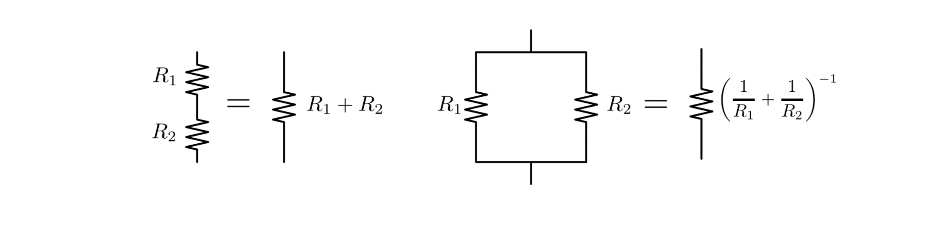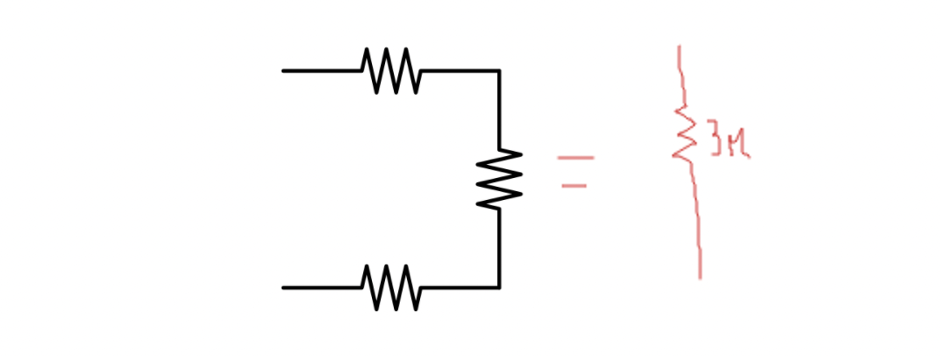top of page
Search
• Yahya Ashraf

# The Infinite Resistance problemAnyone who would have taken an introductory Physics class would have come across this concept of resistors. Resistors are any component of a circuit that provides opposition to current. Represented by a zig-zag line in a circuit, resistors make a really important part of our life. From lamps to street lighting, resistors are everywhere and you would have surely come across one in your physics class. But the problem we are going to discuss today is about an infinite resistor ladder. If we consider an infinitely long chain of resistors, strung together, then what would be the effective resistance? In other words, if you take a circuit with a battery of voltage V and current I and attach an infinitely long chain of resistors then what would be the Effective Resistance, that would show up in Ohm’s law of circuit, V=I * R## How do we calculate the effective resistance in parallel and series circuits?

Before we jump right into the problem, we should first go through some basic rules which are integral for solving this problem. Before we can even tackle this problem, we should know the basics of how we actually find Effective resistance in normal circuits. Throughout this article, I would be using the word “ Effective resistance” so might as well start with defining what I mean by that. Imagine you have a simple circuit with a 2𝛀 resistor and a 1𝛀 resistor so both of these resistors would be contributing to the total resistance of the circuit. The effective resistance of this circuit would be the total resistance contributed by the 2𝛀 and the 1𝛀 resistor. So essentially, we are looking for a resistor with a numerical value of X that would provide the same resistance as the 2𝛀 and 1𝛀 resistor. But how do we find that value of x? Well as it turns out, you just use two formulas, depending on the type of circuit.

There are two types of circuits: parallel and series. In a series circuit, we simply add the resistance ( R1+R2+R2….)The reason is that the same current I is flowing through both resistors, thus the total voltage drop across the pair of them is : VI = IR1 + IR2 = I(R1 + R2) = Reff

However, things are a little trickier in a parallel circuit. In a parallel circuit we add the reciprocal of the resistance and inverse it ((1/R1 + 1/R2 +1/R3…)^-1). This time it's the voltage across each resistor that has to be the same. The current does not have to be equal.Effective resistance in parallel and series

Let's take an example of two very basic parallel and series circuits in order to understand how effective resistance actually varies in these two different arrangements. Imagine that you have two identical resistors connected end to end. So since they are connected in series, the Reff would be simply 2R. Now if you decide to make things a little more complicated and connect these two identical resistors side by side then you have got yourself a parallel circuit. The Reff in this circuit would be (1/R+1/R)^1 which is the same as R/2. Now if you just squint at it and try to observe a pattern then you will realise that the effective resistance is much larger when these resistors are connected in series. This shows a really important property of resistors arranged in parallel and series: in a parallel circuit, the effective resistance will always be less than the resistance of the original resistor and the effective resistance will always be greater than or equal to the original in a series circuit.

## Its all about noticing the pattern

So coming back to the problem. What we want to do is calculate the effective resistance, just like we did for the two resistors, of an infinitely long chain of resistors. Before we jump into mathematics and its concept, it's really important to first ponder over how should we approach this question. Throughout our high school, we have been taught how to put values in a given formula and get the answer but this method only works till it doesn't. Questions like this which tests how well you understand the concept are actually the questions that foster the growth of a mind. This entire question can be easily solved if an individual can identify how the resistors are arranged in this circuit so take a moment and think how you would approach this question.

So first off, we should start by getting an idea of what the answer might be and yes the answer is not infinite. The answer should be somewhat between 2 and 3 but how did I get there? Well, if you take a more careful look then you will realise that the first two resistors are connected in series with the resistors in the box. And with a further careful look, you will realise that inside the box, the third resistor is in parallel with everything else. Earlier we certified that total resistance in parallel is always less than the original resistance so this suggests that the total resistance of the box is less than R. and since that box is in parallel to the first two resistors, the total effective resistance should be between 2 and 3.Now the best way to solve your way through problems involving multiple resistors is to break them down into pieces. So what we can do is break down this circuit into blocks and see what would be the total resistance if we have an infinite number of these three blocks together. The total resistance of the first block would be pretty easy: it's simply 3r.Now if we add two of these blocks together then what would be the effective resistance? Well, this is a much more complex case but we can influence the circuit in such a way so that we end up with a more regular arrangement of series and parallel circuit. We can bend the top left wire so that it is at the red node. From the red node, the current can either take the route with three resistors or the one with one resistor but independent of the route they take, they will meet at the purple node. From there, the current moves towards the green node. Using this information, we can construct a much simpler circuit to help us find effective resistance.So now we have a much simpler arrangement and we simply use our parallel and series circuit equation to find the effective resistance. The math: (⅓ r + 1/r)^-1 + 2r = effective resistance

¾ r + 2r = 11/4r Effective resistance = 11/4 r

To make things more organised, we can denote the number of blocks with a subscript then this is what we have till now: R1= 3r R2=11/4 r

Now what if we added three blocks?Think about adding this third block to the left of our previous two-block circuit. It puts the two-block circuit in parallel with a single resistor R, and then adds on two more resistors in series with that. So instead of calculating the whole three-block resistance from scratch, we can use what we already learned by writingSo now we can simply plug in the value of R2 but the final fraction is not what we want. The thing we wanted to notice is this recursive pattern, that lets us write the resistance for the ladder with N blocks in terms of the resistance RN-1 with one less block, connected up with three more resistors.

So N represents the number of blocks, we can change the above equation slightly to represent the effective resistance of the entire circuit. This is what we get:So what is the value of N in our infinitely long circuit? Well, it's simply ∞. But how do we calculate it when n is ∞. Well, we should first start by realising that ∞-1 is same ∞ so we can substitute N-1 with ∞ itself since adding/removing one more block won't significantly change the effective resistance. Doing the appropriate substitution (substituting n and n-1 with infinity) and then changing the equation to a quadratic equation, this is what we get:Now we can simply solve this equation for R∞ and we will get R∞=(1 + √3 )R. And that's it! That's the effective resistance of an infinitely long chain of resistors, strung together.

This problem is a classical physics problem and it really tests our understanding of the concept. This seemingly hard but actually easy problem could have been easily solved by anyone if they knew how to identify parallel and series arrangements within a circuit and if they were able to see the pattern of arranging different blocks together. Doing these problems periodically really help us get our brains to rinse out the pure concept.

bottom of page